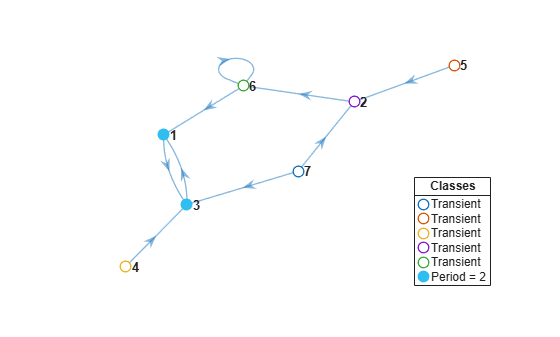# classify

Classify Markov chain states

## Syntax

``bins = classify(mc)``
``[bins,ClassStates,ClassRecurrence,ClassPeriod] = classify(mc)``

## Description

example

``bins = classify(mc)` partitions states of the discrete-time Markov chain `mc` into disjoint communicating classes and returns the class labels `bins` identifying the communicating class to which each state belongs.`

example

``[bins,ClassStates,ClassRecurrence,ClassPeriod] = classify(mc)` additionally returns the states in each class (`ClassStates`), whether the classes are recurrent (`ClassRecurrence`), and class periods (`ClassPeriod`).`

## Examples

collapse all

Consider this theoretical, right-stochastic transition matrix of a stochastic process.

`$P=\left[\begin{array}{cccc}0.5& 0.5& 0& 0\\ 0.5& 0.4& 0.1& 0\\ 0& 0& 0& 1\\ 0& 0& 1& 0\end{array}\right].$`

Create the Markov chain that is characterized by the transition matrix P.

```P = [0.5 0.5 0 0; 0.5 0.4 0.1 0; 0 0 0 1; 0 0 1 0]; mc = dtmc(P);```

Plot a directed graph of the Markov chain. Visually identify the communicating class to which each state belongs by using node colors.

```figure; graphplot(mc,'ColorNodes',true);```States `3` and `4` belong to a communicating class with period 2. States `1` and `2` are transient.

Programmatically identify to which communicating classes the states belong.

`bins = classify(mc)`
```bins = 1×4 1 1 2 2 ```

`bins` is a 1-by-4 vector of communicating class labels. Elements of `bins` correspond to the states in `mc.StateNames`. For example, `bins(3) = 2` indicates that state `3` is in communicating class `2`.

Identify the communicating classes of a Markov chain. Then, determine whether the classes are recurrent and their periodicity.

Generate a random seven-state Markov chain. Specify that 40 random elements in the transition matrix should be zero.

```rng(1); % For reproducibility mc = mcmix(7,'Zeros',40);```

Plot a directed graph of the Markov chain. Visually identify the communicating class to which each state belongs by using node colors.

```figure; graphplot(mc,'ColorNodes',true)```Identify the communicating classes in `mc`, and then determine:

• The communicating class to which each state belongs

• Whether each communicating class is recurrent

• The period of each class

`[bins,ClassStates,ClassRecurrence,ClassPeriod] = classify(mc)`
```bins = 1×7 6 4 6 3 2 5 1 ```
```ClassStates=1×6 cell array {["7"]} {["5"]} {["4"]} {["2"]} {["6"]} {1x2 string} ```
```ClassRecurrence = 1x6 logical array 0 0 0 0 0 1 ```
```ClassPeriod = 1×6 1 1 1 1 1 2 ```

`mc` has seven classes. Each state is its own communicating class, except states `1` and `3`, which together compose class `6`. Class `6` is the only recurrent class; classes 1 through 5 are transient. Class `6` has period `2`; all other classes are aperiodic.

Identify the communicating classes of a Markov chain. Then, extract any recurrent subchains from the Markov chain.

Generate a random seven-state Markov chain. Specify that 40 random elements in the transition matrix should be zero.

```rng(1); % For reproducibility mc = mcmix(7,'Zeros',40);```

Identify all communicating classes in the Markov chain and whether the classes are recurrent.

```[bins,~,ClassRecurrence] = classify(mc); recurrentClass = find(ClassRecurrence,1) ```
```recurrentClass = 6 ```
`recurrentState = find((bins == recurrentClass))`
```recurrentState = 1×2 1 3 ```

Class `6`, composed of states `1` and `3`, is the only recurrent class in `mc`.

Create a subchain from class `6` by specifying at least one state in the subchain. Plot a digraph of the subchain.

```sc = subchain(mc,recurrentState); figure; graphplot(sc,'ColorNodes',true);```## Input Arguments

collapse all

Discrete-time Markov chain with `NumStates` states and transition matrix `P`, specified as a `dtmc` object. `P` must be fully specified (no `NaN` entries).

## Output Arguments

collapse all

Communicating class membership labels for each state, returned as a numeric vector of `NumStates` length. `bins(j)` is the label for the communicating class to which state `j` belongs. Bin values range from 1 through `NumClasses`.

State names in each class, returned as a cell vector of length `NumClasses` containing string vectors. `ClassNames{j}` is the list of state names in class `j`. State names are specified in `mc.StateNames`.

Class recurrence flags, returned as a logical vector of `NumClasses` length.

`ClassRecurrence(j)` indicates whether class `j` is recurrent (`true`) or transient (`false`).

Class periods, returned as a numeric vector of length `NumClasses`. `ClassPeriod(j)` is the period of class `j`. Aperiodic classes have period `1`.

Note

The order of classes in `ClassStates`, `ClassRecurrence`, and `ClassPeriod` corresponds to the class numbers assigned in `bins`.

collapse all

### Communicating Class

Communicating classes of a Markov chain are the equivalence classes formed under the relation of mutual reachability. That is, two states are in the same class if and only if each is reachable from the other with nonzero probability in a finite number of steps.

Communicating classes are equivalent to strongly connected components in the associated digraph . See `graphplot`.

### Irreducible Chain

An irreducible chain is a Markov chain consisting of a single communicating class.

### Unichain

A unichain is a Markov chain consisting of a single recurrent class and any transient classes that transition to the recurrent class.

## Algorithms

• `classify` determines recurrence and transience from the outdegree of the supernode associated with each communicating class in the condensed digraph . An outdegree of 0 corresponds to recurrence; an outdegree that is greater than 0 corresponds to transience. See `graphplot`.

• `classify` determines periodicity using a breadth-first search of cycles in the associated digraph, as in . Class period is the greatest common divisor of the lengths of all cycles originating at any state in the class.

 Gallager, R.G. Stochastic Processes: Theory for Applications. Cambridge, UK: Cambridge University Press, 2013.

 Horn, R., and C. R. Johnson. Matrix Analysis. Cambridge, UK: Cambridge University Press, 1985.

 Jarvis, J. P., and D. R. Shier. "Graph-Theoretic Analysis of Finite Markov Chains." In Applied Mathematical Modeling: A Multidisciplinary Approach. Boca Raton: CRC Press, 2000.